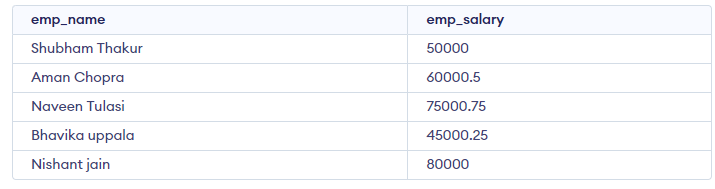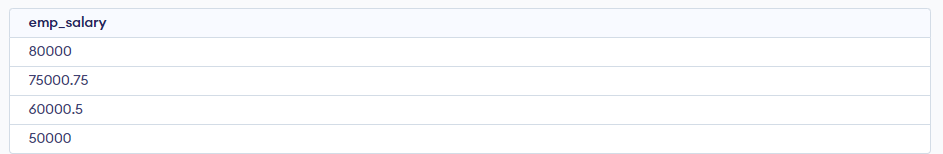GFG App
Open AppBrowser
Continue

# How to find Nth highest salary from a table?

Structured Query Language is a computer language that we use to interact with a relational database. Finding Nth highest salary in a table is the most common question asked in interviews. Here is a way to do this task using the dense_rank() function.

Consider the following table:

Employee:

CREATE TABLE:

```CREATE TABLE emp (
emp_name VARCHAR(50),
emp_salary DECIMAL(10,2)
);```

Let’s insert some random data with a random name and then we will look at how to calculate the nth highest emp_salary.

Query:

```CREATE TABLE emp (
emp_name VARCHAR(50),
emp_salary DECIMAL(10,2)
);
INSERT INTO emp (emp_name, emp_salary) VALUES
('Shubham Thakur', 50000.00),
('Aman Chopra', 60000.50),
('Naveen Tulasi', 75000.75),
('Bhavika uppala', 45000.25),
('Nishant jain', 80000.00);```

Output:Query:

```select * from(
select emp_name, emp_salary, dense_rank()
over(order by emp_salary desc)r from Emp)
where r=3;```
1. To find the 2nd highest sal set n = 2
2. To find the 3rd highest sal set n = 3 and so on.

Let’s check to find 3rd highest salary:

Output:## Using DENSE_RANK

1. DENSE_RANK computes the rank of a row in an ordered group of rows and returns the rank as a NUMBER. The ranks are consecutive integers beginning with 1.
2. This function accepts arguments as any numeric data type and returns NUMBER.
3. As an analytic function, DENSE_RANK computes the rank of each row returned from a query with respect to the other rows, based on the values of the value_exprs in the order_by_clause.
4. In the above query, the rank is returned based on the sal of the employee table. In the case of a tie, it assigns equal rank to all the rows.

## Alternate Solution

```CREATE TABLE `Employee` (
`ENAME` varchar(225) COLLATE utf8_unicode_ci NOT NULL,
`SAL` bigint(20) unsigned NOT NULL,
PRIMARY KEY (`ENAME`)
) ENGINE=InnoDB DEFAULT CHARSET=utf8 COLLATE=utf8_unicode_ci; ```

To find the 6th highest salary

Query:

```mysql> select * from ((select * from Employee
ORDER BY `sal` DESC limit 6 ) AS T)
ORDER BY T.`sal` ASC limit 1;```

## Alternate Use of Limit

```select * from Employee ORDER BY `sal`
DESC limit 5,1; // will return 6th highest ```

Output:## Alternate Solution

Suppose the task is to find the employee with the Nth highest salary from the above table. We can do this as follows:

1. Find the employees with top N distinct salaries.
2. Find the lowest salary among the salaries fetched by the above query, this will give us the Nth highest salary.
3. Find the details of the employee whose salary is the lowest salary fetched by the above query.

Query:

```SELECT * FROM Employee WHERE sal =
(
SELECT MIN(sal) FROM Employee
WHERE  sal IN (
SELECT DISTINCT TOP N
sal FROM Employee
ORDER BY sal DESC
))```

The above query will fetch the details of the employee with the Nth highest salary. Let us see how:

Consider N = 4.

Starting with the most inner query, the query:

Query:

```SELECT DISTINCT sal
FROM Employee
ORDER BY sal DESC
LIMIT 4;```

Output:Query:

```SELECT MIN(sal) FROM Employee WHERE sal IN (
SELECT DISTINCT sal
FROM Employee
ORDER BY sal DESC
LIMIT 4
);```

Output:You can see that the above-returned result is the required 4th highest salary.

Another Solution:

Here N = nth Highest Salary eg. 3rd Highest salary: N=3.

Syntax:

SELECT ename,sal from Employee e1 where

N-1 = (SELECT COUNT(DISTINCT sal)from Employee e2 where e2.sal > e1.sal)

## Using the LIMIT Clause

Syntax:

Select Salary from table_name order by Salary DESC limit n-1,1;

Here we are ordering our salaries in descending order so we will get the highest salary first and then subsequently lower salaries. The limit clause has two components, the First component is to skip a number of rows from the top and the second component is to display the number of rows we want.

Let us see with an example :

To find the 4th Highest salary query will be

Query:

`Select emp_sal from Emp order by emp_sal DESC limit 3,1;`

Output:Here we are skipping 3 rows from the Top and returning only 1 row after skipping.

You can also find names of employees having Nth Highest Salary

Syntax:

Select Emp_name from table_name where Salary =

( Select Salary from table_name order by Salary DESC limit n-1,1);

There can be another question like finding Nth Lowest Salary. In order to do that, just reverse order using ASC ( if you don’t specify by default column will be ordered in ascending order).

Syntax:

Select Salary from table_name order by Salary limit n-1,1;

This article is contributed by Rishav Shandilya. If you like GeeksforGeeks and would like to contribute, you can also write an article using write.geeksforgeeks.org or mail your article to review-team@geeksforgeeks.org. See your article appearing on the GeeksforGeeks main page and help other Geeks.

My Personal Notes arrow_drop_up